﻿ Where is the Border Line between Strong Acids and Weak Acids?

Where is the Border Line between Strong Acids and Weak Acids?

Toratane MunegumiOPEN ACCESSPEER-REVIEWED

Where is the Border Line between Strong Acids and Weak Acids?

Toratane MunegumiDepartment of Science Education, Naruto University of Education, Naruto, Tokushima, Japan

Abstract

The strength of an acid is generally discussed as a unique feature of the acid itself according to its chemical structure. Strong acids have a lower pKa value, and weak acids have a higher one. In the classrooms of high schools and universities, we sometimes use the technical terms “strong acid” and “weak acid”. However, there are some acids for which it is very difficult to say, from their pKa values, whether they are strong or weak. This research addresses the distinction between strong acids and weak acids, in a restricted initial concentration of acids, to provide a clear distinction between strong acids and weak acids.

At a glance: Figures

1
Prev Next

• Munegumi, Toratane. "Where is the Border Line between Strong Acids and Weak Acids?." World Journal of Chemical Education 1.1 (2013): 12-16.
• Munegumi, T. (2013). Where is the Border Line between Strong Acids and Weak Acids?. World Journal of Chemical Education, 1(1), 12-16.
• Munegumi, Toratane. "Where is the Border Line between Strong Acids and Weak Acids?." World Journal of Chemical Education 1, no. 1 (2013): 12-16.

 Import into BibTeX Import into EndNote Import into RefMan Import into RefWorks

1. Introduction

Acid and base are important concepts in chemical education, because they are fundamental concepts that explain features of many chemical compounds. The related concept “neutralization” between acid and base leads to a popular experimental theme, titration, in which students learn about the process and determination of neutralization. During these experiments, students can learn how to use typical glassware, including burettes, pipettes, flasks, etc.

Historically, there are three theories describing the concept of acids and bases : Arrhenius theory, Brønsted-Lowry theory, and Lewis theory. These theories define acids and bases from different viewpoints. The latter two theories include wider concepts and more types of compounds. Therefore, the Brønsted-Lowry theory and the Lewis theory provide wider scope of a generalized view of acids and bases, and are considered more universal than the Arrhenius theory. Donation and acceptance of protons as described by Brønsted-Lowry and donation and acceptance of an electron pair as described by Lewis are very helpful for students and researchers to understand many chemical phenomena. The Brønsted-Lowry theory is particularly useful to understand the strengths of acids and bases from the viewpoint of proton migration. Based on this theory, we can compare many acids and bases using pH, and pKa or pKb values.

Many textbooks [1, 2] explain strong acids as acids having lower pKa values (higher Ka) and weak acids as acids having higher pKa values (lower Ka). This explanation is always correct when comparing one acid with another acid, because it is a relative comparison. However, the representation that one acid is a strong acid, without a relative comparison, sometimes creates some confusion with regard to the strength and weakness of acids.

Although it is easily understandable that hydrochloric acid is a strong acid and acetic acid is a weak acid, it is very difficult to judge whether trifluoroacetic acid is a strong acid or a weak acid. This research discusses an attempt to draw a borderline and to distinguish between strong acids and weak acids.

2. Ka and pKa

2.1. Brønsted-Lowry Theory

Brønsted-Lowry theory defines acids and bases as follows: an acid is any substance that can donate an H+ ion to a base, and a base is any substance that can accept an H+ ion from an acid . Therefore, an acid is a proton donor, and a base is a proton acceptor. Hereafter, when mentioning the terms acid and base, they mean a Brønsted acid and base defined by Brønsted-Lowry theory.

Equations (1) and (2) show the equilibrium of acid and water. In the forward reaction in equation (1), an acid HA donates a proton to a base H2O to give A and H3O+. In the backward reaction, a conjugate base A accepts a proton from a conjugate acid H3O+. In equation (2), the relationship between acid and base is the same as in equation (1).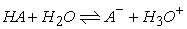(1)(2)

The equilibrium constant Kc and water dissociation constant Kw can be defined as shown in equations (3), (4), and (5). Basically, protons released by an acid do not exist as free protons but as hydronium ions (H3O+) in aqueous solution.(3)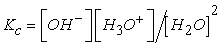(4)(5)

The acid dissociation constant can be defined as shown in equation (6).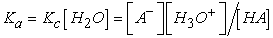(6)

The Ka value is not always suitable for comparing the acidity of many acids, because there are many acids over a wide Ka range. Therefore, use of a logarithm of Ka (pKa) as shown in equation (7) may be better in many cases.(7)

Upon substitution of equation (8), pKa can be represented as shown in equation (9). This is known as the Henderson–Hasselbalch equation.(8)(9)

All acids have their own Ka and pKa values, which are considered to be constant at constant temperature and under other standard conditions. The Henderson–Hasselbalch equation is very important for assigning the strength of acidity. What is the strength of acids?

2.2. Example of Strong and Weak Acids

Generally, if the acid is stronger, the Ka is higher and the pKa is lower. The Ka and pKa of several common acids are listed in Table 1. We can compare the relatively acidity of any two acids by using Ka or pKa.

Table 1. Ka and pKa values of some common of acids (25°C)

However, we sometimes use an absolute assignment of acidity. For instance, an expression such as “this acid is a strong acid and the other is a weak acid” is often used in the field of education. As Table 1 shows, hydroiodic acid, hydrobromic acid, hydrochloric acid, and sulfuric acid have much higher Ka values than the other acids listed, while nitric acid has a lower value, 28.2. These acids are generally classified as strong acids (according to general recognition). However, trifluorocetic acid also seems to be a strong acid. Then where is the borderline between strong acids and weak acids? Before discussing this issue, we must further consider the dissociation and concentration of acids.

3. Degree of Dissociation (α) and pKa

The pKa value does not depend on the concentration of an acid in aqueous solution, but the degree of dissociation does depend on the concentration of acid. The degree of dissociation, which is denoted by the Greek character  (alpha), is defined as shown in equation (10).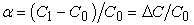(10)

Where C0 is the initial concentration of an acid, C1 is the concentration of the acid at the equilibrium point, and ΔC is the decrease in concentration (from C0).

The value of Ka depends on α, as shown in equation (11).(11)

Therefore, pKa is as shown in equation (12).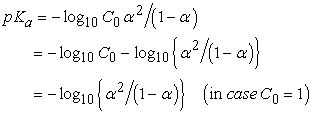(12)

According to this relationship between pKa and α, we obtain a plot of pKa against α for several initial concentrations using MS-Excel as shown in Figure 1.

Figure 1. Relationship between degree of disassociation and pKa of acids in different concentrations of acids at 25°C

Figure 1 shows the relationship between the degree of dissociation and pKa of acids. The seven curves in Fig. 1 compare the degree of dissociation in acid at various (seven) constant concentrations of acids. An acid of pKa = 0 in 1M concentration shows about 10% dissociation, while another acid of pKa = –1.70 in 1M concentration shows about 50% dissociation. Acids that have lower pKa values than about –2.91 and –4 show higher degrees of dissociation than 90% and 99%, respectively. The degrees of dissociation 50%, 90%, and 99% may seem to be the borderline of “most dissociation” at the different concentration levels. Therefore, at 1M, acids of pKa = –1.70, –2.91, and –4 achieve the most dissociation at the levels of 50%, 90%, and 99%, respectively.

The above consideration may suggest a borderline between strong acids and weak acids. For example, in Fig. 1, the point of pKa = –2.91 at 90% dissociation on the curve is a border point. At this point, acids having lower pKa values than –2.91 are strong acids, and acids having higher pKa values than –2.91 are weak acids. However, the curve shifts in parallel to the upper side with the lower initial concentration (C0), and it shifts in parallel to the lower side with the higher initial concentration. The dissociation of acids with pKa = –5 reaches 90% at 10M, and the dissociation of acids with pKa = 1 reaches 90% at 0.0001M.

4. Degree of Dissociation (α) and log10C0for Different Acids

Figure 2 shows the relationship between the degree of dissociation (α) and log10C0 for several acids. The plots are obtained from picked up values for pKa of each acid in Figure 1.

The degree of dissociation at log10C0=0 is due to that at C0=1 (M). Comparing degree of dissociation of some acids, we can say which is strong or weak acid. Hydrochloric acid (pKa= -6) dissociates almost 100%. Sulfuric acid (pKa= -3), hydronium ion (pKa= -1.74), nitric acid (pKa= -1.45), trifluoroacetic acid (pKa= 0.25), oxalic acid (pKa= 1.27) dissociate 92, 52, 41, 7.2, and 2.3%, respectively. Border is a virtual acid with pKa = 0 (Ka =1).

The lower the concentration, the higher the degree of dissociation of all these acids. At log10C0 = -3, these acids show higher degree of dissociation than 50%. However, acetic acid (pKa=4.76) does not dissociate even at this point.

5. Distinction between Strong Acid and Weak Acid in Textbooks

5.1. Some Textbooks for High School in Japan

In order to consider the explanation of strong and weak acids commonly expounded by teachers, the description given in four Japanese textbooks [4, 5, 6, 7] published by different publishers, for use in high schools, is now mentioned.

(1) ‘High School Chemistry IB’ (one B), published by Jikkyo Shuppan, gives the following description: “Acids like hydrochloric acid, nitric acid and sulfuric acid have a higher degree of dissociation to give many H+ ions. Therefore, these are strong acids showing a high acidity.”

(2) ‘Shintei Chemistry IB’, published by Dainippon Tosho, gives the following description: “Acids possessing a degree of dissociation near one are strong acids. For example, hydrochloric acid, nitric acid and sulfuric acid are strong acids. Acids possessing a degree of dissociation much lower than one are weak acids”. By the degree of dissociation one means 100% dissociation .

(3) ‘Shosetsu Chemistry IB’, published by Sanseido, gives the following description: “Generally, acids showing a degree of dissociation near one are strong acids, and acids showing a low degree of dissociation are weak acids” .

(4) ‘High School Chemistry I’ published by Keirinsha gives the following description: “Strong acids show a degree of dissociation near one, even at high concentration, and weak acids show an extremely lower degree of dissociation than one”. However, they explain the degree of dissociation as follows, for example: “Degree of dissociation is different from variety of substances, for instance, hydrochloric acid possesses degree of dissociation one and acetic acid possesses extremely lower degree of dissociation than 1. Moreover, the degree of dissociation changes according to concentration and temperature. Generally, the lower the concentration the higher the degree and the higher the temperature the higher the degree” .

Examples in the textbooks 1–3 above [4, 5, 6] do not mention the dependency of the degree of dissociation on the concentration of acids, but the example in textbook 4  does mention it. Distinguishing between strong acids and weak acids according to the degree of dissociation without considering the condition involving concentration is incorrect, because all acids can show 100% dissociation at infinite dilution. In this case, nobody distinguishes between strong acids and weak acids.

The educational items that can be taught in high school have been restricted by curriculum guidelines. Nonetheless, correct explanations must be given. It is my hope that the concept of pKa will be taught in high school. If so, students would understand the difference between strong and weak acids, without a gap between high school and university.

5.2. Some Textbooks for Universities in USA

The examples of two English textbooks that have been published for use in universities in the USA and have been translated into Japanese are now mentioned. ‘Chemistry: structure and dynamics, 5th ed.’, written by Spencer et al., distinguishes between strong acids and weak acids based on Ka as shown in Table 2 .

Table 2. Strong acid and weak acid, as described by Spencer et al. (25°C)

Classification using pKa in Table 2 is based on the value calculated from the distinction based on Ka. Appling this distinction rule to the acids listed in Table 1, we can segregate strong acids and weak acids. Strong acids include the following: hydroiodic acid, hydrobromic acid, hydrochloric acid, sulfuric acid, the hydroxonium ion, and nitric acid. Weak acids include the other acids listed.

Although the distinction rule used by Spencer et al. is very clear, there are some shortcomings between this rule and the general perception [9, 10]. Trifluoroacetic acid, distinguished as a weak acid by this rule, has been recognized as a strong acid by organic chemists [9, 10]. We can now wonder whether such a very clear distinction is useful or not. Furthermore, as shown in Figure 1, even a 1M acid with pKa=0 (Border in Figure 2) gives only 9.5% degree of dissociation. Not surprisingly, such a low dissociation fits the general perception of acid. In the distinction of acids, their concentration may be considered as a factor.

On the other hand, ‘Organic chemistry, 5th ed.’, written by Bruice, distinguishes between strong acids and weak acids as shown in Table 3 .

Table 3. Strong acid and weak acid, as described by Bruice (25°C)

According to the distinction in Table 3, trifluoroacetic acid (pKa = 0.25), oxalic acid (pKa = 1.27), and acetic acid (pKa = 4.76) are classified in the category “very strong acid”, “moderately strong acid”, and “weak acid,” respectively. This distinction may meet the general perception of organic chemists. The reason for this distinction is, however, not clearly shown.

6. Proposal of an Appropriate Distinction between Strong Acids and Weak Acids

As has been explained above, for the distinction between strong acids and weak acids, the concentration of acids is required, as a reference point, when considering the degree of dissociation. We have noted incorrect representation in some Japanese high school textbooks: they do not use pKa, they only use the degree of dissociation. The use of pKa for distinguishing the strength of acids in some university textbooks is appropriate, because pKa does not depend on the concentration of acids. However, in high school textbooks, the use of pKa for the distinction between strong acids and weak acids has to be clearly explained to students.

I would like to propose a more appropriate distinction between strong acids and weak acids. See Fig. 3. The curve in Fig. 3 shows a relationship between the degree of dissociation of acid and pKa in 0.0001M acid. There are four regions: a curve in the lower range of pKa 1 (very strong acid), an almost linear curve in the rage of pKa 1–3 (moderately strong acid), a curve in the range of pKa 3–5 (weak acid), and a curve in the higher range of pKa 5 (very weak acid). This distinction is an application of Bruice’s concept to the curve at the concentration of 0.0001M in Fig. 1. Bruice’s distinction in Table 2 seems to meet the perception of organic chemists.

However, the distinction in Figure 3 gives adequate reason for the distinction. In the region of very strong acid, the pKa rapidly decreases with a decrease in the degree of dissociation (100-90%). In the region of moderately strong acid, the pKa linearly and moderately decreases with a decrease in the degree of dissociation (90-30%). In the region of weak acid, pKa rapidly decreases with a decrease in the degree of dissociation (30-3%). In the region of very weak acid, pKa decreases very rapidly.

Figure 3. Explanation of distinction between strong acids and weak acids

7. Conclusions

The proposed distinction of acids by pKa is not in conflict with the perception of organic chemists, and it is appropriate to explain strong acids and weak acids to students, together with the degree of dissociation.

References

  Spencer, J.N., Bodner, G.M., Rickard, L.H., Chemistry structure and dynamics, 5th ed., John Wiley & Sons, Inc., Hoboken, NJ, USA, 2012.In article  Bruice, P.Y., Organic Chemistry, 5th ed., Pearson Education Inc. publishing as Prentice Hall, Upper Saddle River, NJ, USA, 2006, 46.In article  Spencer, J.N., Bodner, G.M., Rickard, L.H., Chemistry structure and dynamics, 5th ed., John Wiley & Sons, Inc., Hoboken, NJ, USA, 2012, 471.In article  "High school Chemistry IB", p90, Jikkyo Shuppan, (1995).In article  "Shintei Chemistry IB", p80, Dainippon Tosho (1997).In article  "Shosetsu Chemistry IB", p114, Sanseido (1997).In article  "High School Chemistry I", p88, Keirinkan (2006).In article  Spencer, J.N., Bodner, G.M., Rickard, L.H., Chemistry structure and dynamics, 5th ed., John Wiley & Sons, Inc., Hoboken, NJ, USA, 2012, 484.In article  Zhao, H., Holladay, J.E., Kwak, J.H. and Zhang, Z.C., "Inverse temperature-dependent pathway of cellulose decrystallization in trifluoroacetic acid," J. Phys. Chem. B, 111 (19). 5295-5300. Oct. 2007.In article CrossRef PubMed  Eisenbach, C.D. and Sperlich, B., "Copolymerization of acrylonitrile and ethane in the presence of trifluoroacetic acid as complexing agent," Macromolecule, 29 (24). 7748-7752. Dec. 1996.In article CrossRef